﻿ 高温作业专用服装的研究 The Research of Special Clothing for High Temperature Operation

Vol. 07  No. 12 ( 2018 ), Article ID: 28151 , 10 pages
10.12677/AAM.2018.712185

The Research of Special Clothing for High Temperature Operation

Yuting Wang, Haoran Qin, Guanghao Zhang, Kaisen Zhang

Shenyang Aerospace University, Shenyang Liaoning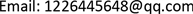Received: Nov. 23rd, 2018; accepted: Dec. 17th, 2018; published: Dec. 24th, 2018ABSTRACT

In this paper, based on the design problem of high-temperature special clothing, the lumped parameter method and double-layer glass heat transfer model are used to establish the temperature distribution model, optimization model and temperature difference model of garment materials. Using MATLAB, LINGO and other software to solve the model, the relationship between temperature, time function and the optimal thickness of fabric material are obtained. Considering that the heat transfer method only considers the heat conduction, the compensation coefficient is defined to reduce the error of the model. This model provides a reliable basis for the design and cost control of special clothing for high temperature operation. This paper can also be used to predict the insulation effect of insulating glass and the insulation effect of buildings in winter. The accuracy of this paper is higher and the prediction results are accurate.

Keywords:Special Clothing for High Temperature Operation, Lumped Parameter Method, Difference Methods, Optimization1. 背景知识

1.1. 背景介绍

1.2. 研究意义

2. 问题的分析

2.1. 对环境温度为75℃、II层厚度为6 mm、IV层厚度为5 mm，研究人皮肤外侧的温度分布

2.2. 当环境温度为65℃、IV层的厚度为5.5 mm时，确定II层的最优厚度， 确保工作60分钟时，不超过人体承受范围

2.3. 当环境温度为80℃时，确定II层和IV层的最优厚度，确保工作30分钟时， 假人皮肤外侧温度不超过47℃，且超过44℃的时间不超过5分钟

1、假设热传导是唯一的传热方式；

2、假设材料的厚度为其特征尺寸；

3、织物材料无褶皱且表面温度处处相等；

4、假设温度传递只存在一维传递且沿着温度梯度方向；

5、假设防护服的表面积与人体的表面积相等，均为1.6 m2

6、假设材料的热传导率与表面传感系数在数值上相等；

7、假设不存在导热热阻；

8、假设第III、IV层温度为衣服温度 ${y}_{0}=30˚\text{C}$ ；第I、II层温度为体内温度 ${y}_{体}=37˚\text{C}$

3. 模型的建立与求解

3.1. 环境温度75℃时，关于服装温度变化的研究

3.1.1. 研究思路

3.1.2. 研究方法

1) 模型原理

$Q=\lambda \frac{\Delta T}{d}$

2) 模型建立

$Q={\lambda }_{1}\frac{{y}_{1}-{y}_{\infty }}{{d}_{1}}={\lambda }_{2}\frac{{y}_{2}-{y}_{1}}{{d}_{2}}={\lambda }_{3}\frac{{y}_{3}-{y}_{2}}{{d}_{3}}={\lambda }_{4}\frac{{y}_{4}-{y}_{3}}{{d}_{4}}$

3) 模型数据处理

${y}_{1}=71˚\text{C}$

${y}_{2}=62.13˚\text{C}$

${y}_{3}=57.77˚\text{C}$

1) 模型的建立

$\frac{y-{y}_{\infty }}{{y}_{0}-{y}_{\infty }}=\mathrm{exp}\left(-\frac{hA}{\rho cV}x\right)$

$\rho$ ——表示传热物质密度；

①方程的整理：

$y=\left({y}_{0}-{y}_{\infty }\right)\mathrm{exp}\left(-g\frac{hA}{\rho cV}x\right)+{y}_{\infty }$

${y}_{1}=\left({y}_{0}-{y}_{\infty }\right)\mathrm{exp}\left(-1.35\frac{hA}{\rho cV}x\right)+75$

${y}_{2}=\left({y}_{0}-{y}_{1}\right)\mathrm{exp}\left(-10.44\frac{hA}{\rho cV}x\right)+{y}_{1}$

${y}_{3}=\left({y}_{体}-{y}_{2}\right)\mathrm{exp}\left(-3.95\frac{hA}{\rho cV}x\right)+{y}_{2}$

${y}_{4}=\left({y}_{体}-{y}_{3}\right)\mathrm{exp}\left(-0.15\frac{hA}{\rho cV}x\right)+{y}_{3}$

3.2. 环境温度65℃时，第二层最优厚度的研究

3.2.1. 研究思路

3.2.2. 研究方法

1) 模型准备

2) 模型的建立

$m<12\text{\hspace{0.17em}}\text{kg}$

$m={m}_{\text{I}}+{m}_{\text{II}}+{m}_{\text{III}}+{m}_{\text{IV}}$

$0.0006\text{\hspace{0.17em}}\text{m}<{d}_{2}<0.025\text{\hspace{0.17em}}\text{m}$

${y}_{21}=65˚\text{C}$

${\lambda }_{1}\frac{{y}_{1}-{y}_{2}}{{d}_{1}}>{\lambda }_{2}\frac{{y}_{21}-{y}_{22}}{{d}_{21}}$

${y}_{21}-{y}_{22}<8.87$

${g}_{4}=0.15$

${\lambda }_{1}\frac{{y}_{21}-{y}_{22}}{{d}_{1}}={\lambda }_{2}\frac{{y}_{22}-{y}_{23}}{{d}_{2}}={\lambda }_{3}\frac{{y}_{23}-{y}_{24}}{{d}_{3}}={\lambda }_{4}\frac{{y}_{23}-47}{{d}_{4}}$

$x=55\text{\hspace{0.17em}}\mathrm{min}$ 时假人皮肤外侧温度不超过44℃

$44\ge \left({y}_{体}-{y}_{x=55}\right)\mathrm{exp}\left(-{g}_{4}\frac{hA}{\rho cV}\right)+{y}_{x=55}$

$47\ge \left({y}_{体}-{y}_{23}\right)\mathrm{exp}\left(-{g}_{4}\frac{hA}{\rho cV}\right)+{y}_{23}$

${y}_{x=55}>44˚\text{C}$

${y}_{23}>47˚\text{C}$

3) 模型的求解

${d}_{2}=\text{8}\text{.17}\text{\hspace{0.17em}}\text{mm}$ 代入问题研究一模型中。在60分钟时，可以确定各层的温度如表1所示。Table 1. Temperature form of each layer in 60 minutes

3.3. 当环境温度为80℃，确定II层和IV层的最优厚度

3.3.1. 研究思路

3.3.2. 研究方法

1) 模型原理与建立

$\frac{\partial {y}_{i}}{\partial {x}_{i}}=\frac{{\lambda }_{i}}{{c}_{i}{\rho }_{i}}\frac{{\partial }^{2}{y}_{i}}{\partial {l}^{2}}$

${\lambda }_{i}\frac{\partial {y}_{i}}{\partial x}={\lambda }_{i+1}\frac{\partial {y}_{i+1}}{\partial x},{y}_{i}={y}_{i+1},l={d}_{i},\left(i=1,2,3,4\right)$

$\left\{\begin{array}{l}\frac{\partial {y}_{i}}{\partial x}=\frac{{\lambda }_{i}}{{c}_{i}{\rho }_{i}}\frac{{\partial }^{2}{y}_{i}}{\partial {l}^{2}}\\ {\lambda }_{i}\frac{\partial {y}_{i}}{\partial l}={\lambda }_{i+1}\frac{\partial {y}_{i+1}}{\partial l},{y}_{i}={y}_{i+1},l={d}_{i},\left(i=1,2,3\right)\\ {y}_{0}\left(0,x\right)=80˚\text{C}\\ {y}_{4}\left({L}_{4},x\right)={y}_{0}=37˚\text{C}\end{array}$

2) 模型求解

x——时间；d(Y)——厚度(空间变量)；U——温度；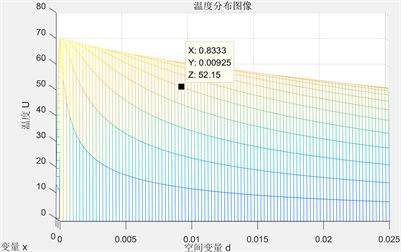Figure 1. The II layer relationship of temperature distribution to thickness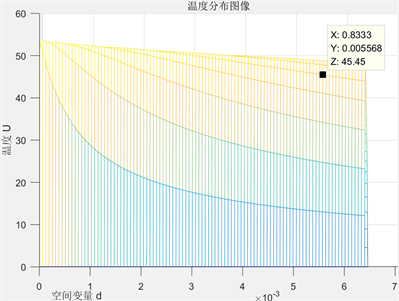Figure 2. The IV layer relationship of temperature distribution to thickness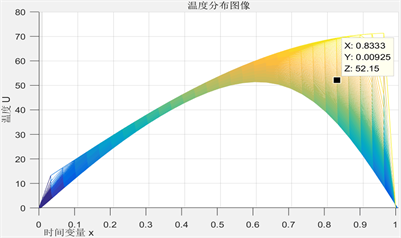Figure 3. The II layer relationship of temperature distribution to time variable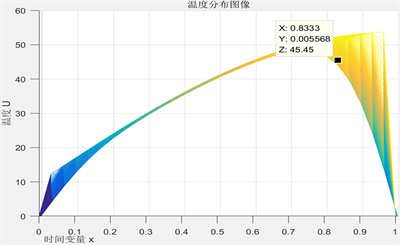Figure 4. The IV layer relationship of temperature distribution to time variable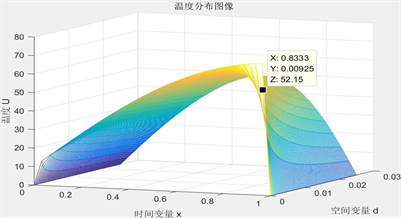Figure 5. The II layer relationship of temperature distribution, time variable and thickness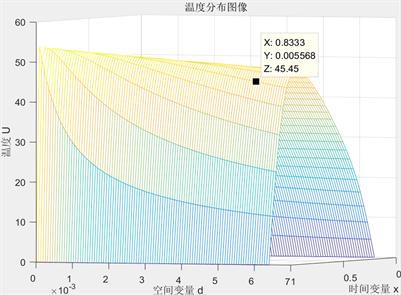Figure 6. The IV layer relationship of temperature distribution, time variable and thickness

4. 结论

The Research of Special Clothing for High Temperature Operation[J]. 应用数学进展, 2018, 07(12): 1583-1592. https://doi.org/10.12677/AAM.2018.712185

1. 1. 华涛. 热防护服热防护性能的分析与探讨[J]. 产业用纺织品, 2002(8): 28-31.

2. 2. 李俊, 等. 耐高温防护服及其发展趋势[EB/OL]. 百度文库. https://wenku.baidu.com/view/5214ac6a2f3f5727a5e9856a561252d380eb2018.html?tdsourcetag=s_pctim_aiomsg&qq-pf-to=pcqq.group

3. 3. 戴锅生. 传热学[M]. 第2版. 北京: 高等教育出版社, 1999.

4. 4. 李庆杨, 王能超, 易大义. 数值分析[M]. 第5版. 北京: 清华大学出版社, 2008.

5. 5. 百度文库. 永冻土层热传导问题数学建模[EB/OL]. https://wenku.baidu.com/view/4cdf33421a37f111f0855bab.html